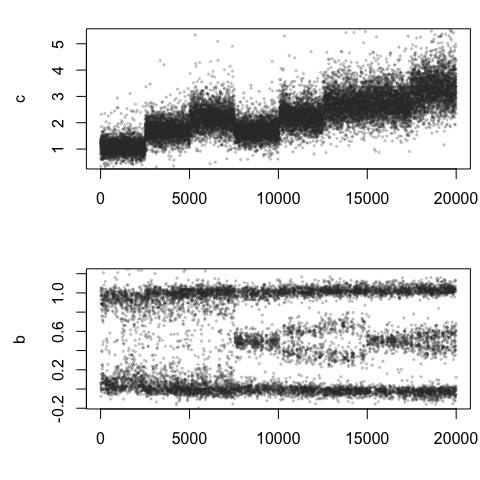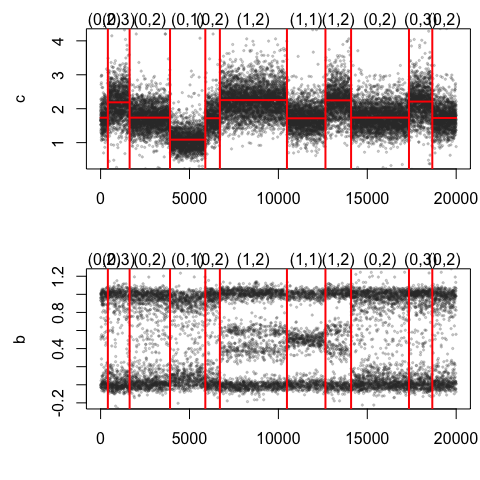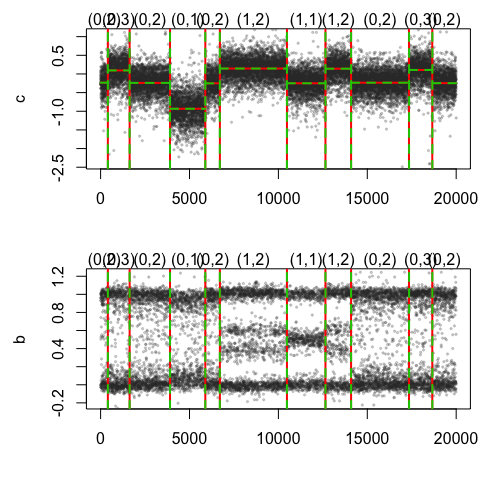# PSSeg: Parent-Specifc copy number segmentation

#### 2019-01-11

This vignette describes how to use the jointseg package to partition bivariate DNA copy number signals from SNP array data into segments of constant parent-specific copy number. We demonstrate the use of the PSSeg function of this package for applying two different strategies. Both strategies consist in first identifying a list of candidate change points through a fast (greedy) segmentation method, and then to prune this list is using dynamic programming . The segmentation method presented here is Recursive Binary Segmentation (RBS, ). We refer to  for a more comprehensive performance assessment of this method and other segmentation methods. segmentation, change point model, binary segmentation, dynamic programming, DNA copy number, parent-specific copy number.

Please see Appendix for citing jointseg.

## Preparing data to be segmented

PSSeg requires normalized copy number signals, in the form of total copy number estimates and allele B fractions for tumor, the (germline) genotype of SNP. Loci are assumed to come from a single chromosome and to be ordered by genomic position.

For illustration, we show of such a data set may be created from real data. We use data from a public SNP array data set, which is distributed in the acnr package (on which the jointseg package depends).

data <- acnr::loadCnRegionData(dataSet="GSE29172", tumorFraction=1)
str(data)
## 'data.frame':    40000 obs. of  7 variables:
##  $c : num 0.909 0.859 1.304 0.647 0.947 ... ##$ b          : num  NaN NaN NaN NaN NaN NaN NaN -0.035 NaN NaN ...
##  $genotype : num NA NA NA NA NA NA NA 0 NA NA ... ##$ bT         : num  NaN NaN NaN NaN NaN NaN NaN 0.161 NaN NaN ...
##  $bN : num NaN NaN NaN NaN NaN NaN NaN 0.196 NaN NaN ... ##$ region     : chr  "(0,1)" "(0,1)" "(0,1)" "(0,1)" ...
##  $cellularity: num 1 1 1 1 1 1 1 1 1 1 ... This data set consists of copy number signals from 8 types of genomic regions: table(data[["region"]]) ## ## (0,1) (0,2) (0,3) (1,1) (1,2) (1,3) (2,2) (2,3) ## 5000 5000 5000 5000 5000 5000 5000 5000 These regions are coded as $$(C_1,C_2)$$, where $$C_1$$ denotes the minor copy number and $$C_2$$ denotes the major copy number, i.e. the smallest and the largest of the two parental copy numbers (see e.g.  for more detailed definitions). For example, $$(1,1)$$ corresponds to a normal state, $$(0,1)$$ to an hemizygous deletion, $$(1,2)$$ to a single copy gain and $$(0,2)$$ to a copy-neutral LOH (loss of heterozygosity). idxs <- sort(sample(1:nrow(data), 2e4)) plotSeg(data[idxs, ])These real data can then be used to create a realistic DNA copy number profile of user-defined length, and harboring a user-defined number of breakpoints. This is done using the getCopyNumberDataByResampling function. Breakpoint positions are drawn uniformly) among all possible loci. Between two breakpoints, the copy number state corresponds to one of the types of regions in data}, and each data point is drawn with replacement from the corresponding true copy number signal from the region. More options are available from the documentation ofgetCopyNumberDataByResampling}. K <- 10 bkp <- c(408,1632,3905, 5890,6709, 10481, 12647,14089,17345,18657) len <- 2e4 sim <- getCopyNumberDataByResampling(len, bkp=bkp, minLength=500, regData=data) datS <- sim$profile
str(datS)
## 'data.frame':    20000 obs. of  7 variables:
##  $c : num 1.92 1.66 1.67 1.74 1.66 ... ##$ b          : num  -0.005 NaN 0.933 NaN NaN NaN 0.961 0.959 NaN NaN ...
##  $genotype : num 0 NA 1 NA NA NA 0.5 1 NA NA ... ##$ bT         : num  0.041 NaN 0.699 NaN NaN NaN 0.966 0.776 NaN NaN ...
##  $bN : num 0.046 NaN 0.766 NaN NaN NaN 0.56 0.817 NaN NaN ... ##$ region     : chr  "(0,2)" "(0,2)" "(0,2)" "(0,2)" ...
##  $cellularity: num 1 1 1 1 1 1 1 1 1 1 ... The resulting copy-number profile is plotted below. plotSeg(datS, sim$bkp)## Preprocessing

We advise the following (typical) preprocessing before segmentation: * $$\log$$-transform total copy numbers in order to stabilize their variance; this step improve segmentation results for all methods.

datS$c <- log2(datS$c)-1
• smooth single point outliers as suggested by  This step is controlled by the dropOutliers option in the PSSeg function, which internally calls the smooth.CNA function of the DNAcopy package. The default value for this option is TRUE.
• convert allelic ratios to (unimodal) decrease in heterozygosity ($$d$$), as initially suggested by . This step is performed internally in the PSSeg function.

## PSSeg segmentation using RBS

We can now use the PSSeg function to segment signals. The method consists in three steps:

• run a fast (yet approximate) segmentation on these signals in order to obtain a set of (at most hundreds of) candidate change points. This is done using Recursive Binary Segmentation (RBS );
• prune the obtained set of change points using dynamic programming 
• select the best number of change points using a model selection criterion proposed by 

## Initial segmentation and pruning

resRBS <- PSSeg(data=datS, K=2*K, method="RBS", stat=c("c", "d"), profile=TRUE)

Note that this is fast:

resRBS$prof[, "time"] ## segmentation dpseg ## 0.06 0.00 ## Plot segmented profile To plot the PSSeg segmentation results together with the true breakpoints, do : plotSeg(datS, list(true=sim$bkp, est=resRBS$bestBkp))## Results evaluation The PSSeg function returns the original segmentation (by RBS), the result of the pruning step, and the best model (among those selected by dynamic programming) according to the criterion proposed by . The quality of the best segmentation can be assessed as follows. The number of true positives (TP) is the number of true change points for which there exists a candidate change point closer than a given tolerance tol. The number of false positives is defined as the number of true negatives (all those which are not change points) for which the candidate change points are out of tolerance area and those in tolerance area where there already exists a candidate change point. %The true negative rate (TNR) is defined as 1-FPR. % True negative are defined as the midpoints of intervals between true change points (augmented by points 0 and $$n+1$$, where $$n$$ is the number of loci. The true negative rate (TNR) is the proportion of true negatives for which there is no candidate change point closer than tol}. By construction, $$TP \in \{0, 1, \cdots, K \}$$ where $$K$$ is the number of true change points. print(getTpFp(resRBS$bestBkp, sim$bkp, tol=5)) ## TP FP ## 9 1 Obviously, this performance measure depends on the chosen tolerance: perf <- sapply(0:10, FUN=function(tol) { getTpFp(resRBS$bestBkp, sim\$bkp, tol=tol,relax = -1)
})
print(perf)
##    [,1] [,2] [,3] [,4] [,5] [,6] [,7] [,8] [,9] [,10] [,11]
## TP    4    7    7    9    9    9   10   10   10    10    10
## FP    6    3    3    1    1    1    0    0    0     0     0

## Session information

sessionInfo()
## R version 3.5.1 (2018-07-02)
## Platform: x86_64-apple-darwin15.6.0 (64-bit)
## Running under: OS X El Capitan 10.11.6
##
## Matrix products: default
## BLAS: /Library/Frameworks/R.framework/Versions/3.5/Resources/lib/libRblas.0.dylib
## LAPACK: /Library/Frameworks/R.framework/Versions/3.5/Resources/lib/libRlapack.dylib
##
## locale:
##  C/fr_FR.UTF-8/fr_FR.UTF-8/C/fr_FR.UTF-8/fr_FR.UTF-8
##
## attached base packages:
##  stats     graphics  grDevices utils     datasets  methods   base
##
## other attached packages:
##  jointseg_1.0.2 knitr_1.20
##
## loaded via a namespace (and not attached):
##   Rcpp_1.0.0         matrixStats_0.54.0 digest_0.6.18
##   rprojroot_1.3-2    acnr_1.0.0         backports_1.1.2
##   magrittr_1.5       evaluate_0.12      stringi_1.2.4
##  rmarkdown_1.10     tools_3.5.1        stringr_1.3.1
##  yaml_2.2.0         compiler_3.5.1     htmltools_0.3.6
##  DNAcopy_1.54.0

## Citing jointseg

citation("jointseg")
##
## To cite package 'jointseg' in publications, please use the
## following references:
##
##   Morgane Pierre-Jean, Guillem Rigaill and Pierre Neuvial ().
##   jointseg: Joint segmentation of multivariate (copy number)
##   signals.R package version 1.0.2.
##
##   Morgane Pierre-Jean, Guillem Rigaill and Pierre Neuvial.
##   Performance evaluation of DNA copy number segmentation methods.
##   Briefings in Bioinformatics (2015) 16 (4): 600-615.
##
## To see these entries in BibTeX format, use 'print(<citation>,
## bibtex=TRUE)', 'toBibtex(.)', or set
## 'options(citation.bibtex.max=999)'.`